## 2.20Natural convection

In Sec. 2.13 , a set of equations — Eq. (2.47 ) and Eq. (2.48 ) — was derived for ﬂow of an incompressible ﬂuid. They are a sample set of equations for mass and momentum conservation that can be solved using methods described in this book.

The example set of equations can be extended to include energy conservation and associated models of heat conduction and heat capacity, described in Sec. 2.15  - 2.18 .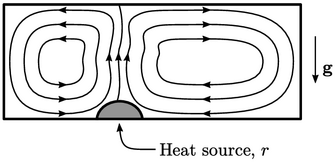The set of equations for mass, momentum and energy can be combined to simulate natural convection, e.g. for ﬂow of air around a room. In natural convection, a non-uniform temperature causes density variations which generate associated forces due to gravity. Colder air is driven downwards and hot air rises, creating buoyancy. Small temperature variations, e.g. due to a heat source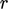, can cause buoyancy to be the dominant force.

A simple, approximate equation for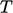can be derived, starting from internal energy conservation in the form of Eq. (2.57 ). The approximations of constant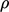(with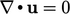) and zero viscosity reduce the stress/pressure work terms to zero.

Assuming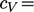constant, we can apply Eq. (2.63 ), which reduces to substituting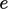by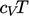since derivatives of constants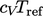and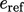are zero. Applying Fourier’s law Eq. (2.54) leads to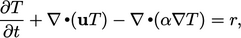(2.65)
where thermal diﬀusivity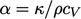and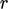becomes a thermal source in SI units of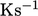.

This is another example, similar to Eq. (2.49 ), of a transport equation containing a time derivative, advection, diﬀusion and a source of heat. Applying suitable boundary conditions, the equation can be solved for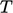.

### Buoyancy force

The eﬀect of buoyancy can be simulated by setting the body force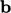in Eq. (2.47 ) for an incompressible, Newtonian ﬂuid. While the assumption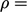constant is applied across all the governing equations generally, it cannot be applied to this force. Therefore we apply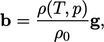(2.66)
where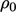is a density at a reference state, e.g. at the initialand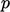, andis the acceleration due to gravity.

The density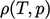is a function ofand, optionally,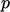provided by some equation of state, e.g. the ideal gas Eq. (2.55 ). The ﬁnal momentum equation including this buoyancy force, and assuming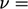constant is: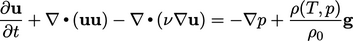(2.67)
The combined set of equations for mass, momentum and energy becomes Eq. (2.48 ) Eq. (2.67 ) and Eq. (2.65 ) respectively, which can be used to solve ﬂows with natural convection.
Notes on CFD: General Principles - 2.20 Natural convection